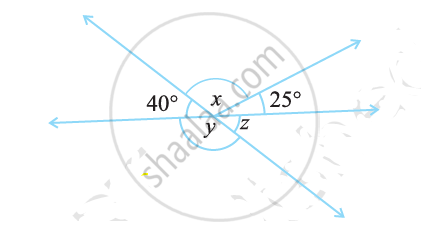Share

# Find the Value of the Angles X, Y, And Z In the Following:2 - CBSE Class 7 - Mathematics

ConceptConcept of Vertically Opposite Angles

#### Question

Find the value of the angles xy, and z in the following:#### Solution

z = 40° (Vertically opposite angles)

y + ∠z = 180° (Linear pair)

y = 180° − 40° = 140°

40° + ∠+ 25° = 180° (Angles on a straight line)

65° + ∠= 180°

x = 180° − 65° = 115°

Is there an error in this question or solution?

#### APPEARS IN

NCERT Solution for Mathematics for Class 7 (2018 to Current)
Chapter 5: Lines and Angles
Ex. 5.10 | Q: 12.2 | Page no. 103
Solution Find the Value of the Angles X, Y, And Z In the Following:2 Concept: Concept of Vertically Opposite Angles.
S